## 4.1.4 Spectral quantization

06.203GPPHalf rate speech transcodingTS

A three segment vector quantizer of the reflection coefficients is employed. A reduced complexity search technique is used to select the vector of reflection coefficients for each segment. The reflection coefficient vector quantizer codebooks are stored in compressed form to minimize their memory requirements.

The three segments of the vector quantizer span reflection coefficients r1  r3, r4  r6, and r7 – r10 respectively. The bit allocations for the vector quantizer segments are:

 Q1 11 bits Q2 9 bits Q3 8 bits

A reflection coefficient vector prequantizer is used at each segment. The prequantizer size at each segment is:

 P1 6 bits P2 5 bits P3 4 bits

At a given segment, the residual error due to each vector from the prequantizer is computed and stored in temporary memory. This list is searched to identify the four prequantizer vectors which have the lowest distortion. The index of each selected prequantizer vector is used to calculate an offset into the vector quantizer table at which the contiguous subset of quantizer vectors associated with that prequantizer vector begins. The size of each vector quantizer subset at the k-th segment is given by: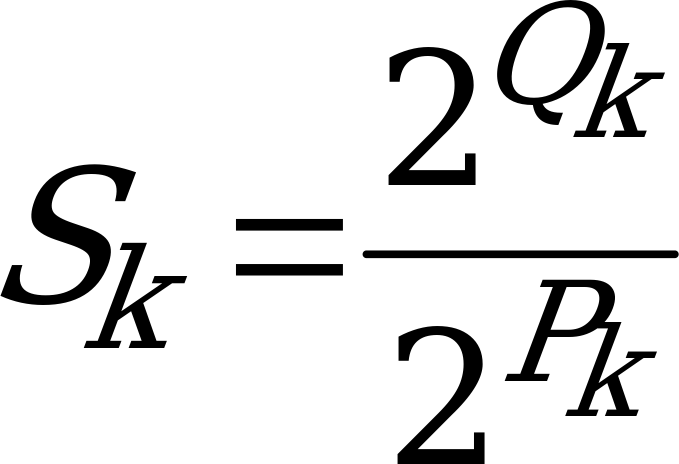(14)

The four subsets of quantizer vectors, associated with the selected prequantizer vectors, are searched for the quantizer vector which yields the lowest residual error. Thus at the first segment, 64 prequantizer vectors and 128 quantizer vectors are evaluated, 32 prequantizer vectors and 64 quantizer vectors are evaluated at the second segment, and 16 prequantizer vectors and 64 quantizer vectors are evaluated at the third segment.

#### 4.1.4.1 Autocorrelation Fixed Point Lattice Technique (AFLAT)

An autocorrelation version of the FLAT algorithm, AFLAT, is used to compute the residual error energy for a reflection coefficient vector being evaluated. Compute the autocorrelation sequence R(i), from the optimal reflection coefficients, rj, over the range 0 £ i £ Np.

 STEP 1 Define the initial conditions for the AFLAT recursion: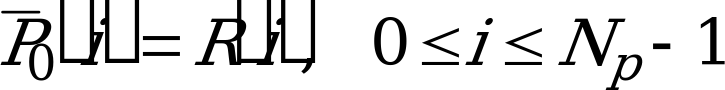(15)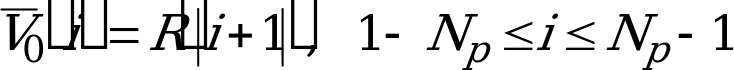(16) STEP 2 Initialize k, the vector quantizer segment index:k = 1 (17) STEP 3 Let Il(k) be the index of the first lattice stage in the k-th segment, and Ih(k) be the index of the last lattice stage in the k-th segment. STEP 4 Initialize j, the index of the lattice stage, to point to the beginning of the k-th segment:EMBED Equation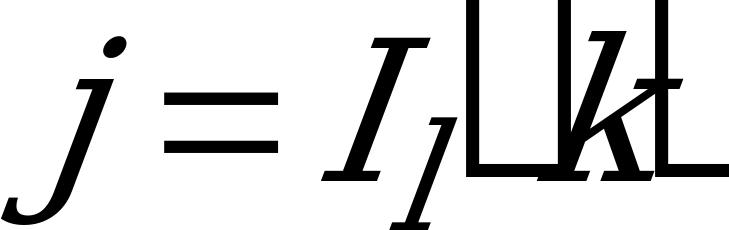(18) STEP 5 Set the initial conditions Pj‑1 and Vj‑1 to: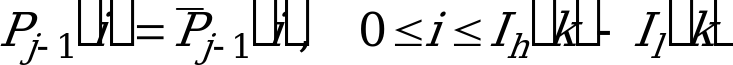(19)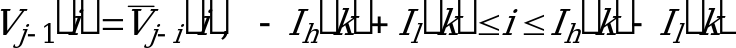(20) STEP 6 Compute the values of Vj and Pj arrays using: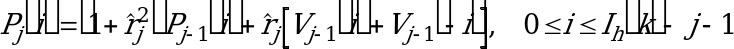(21)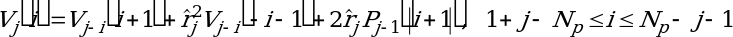(22) STEP 7 Increment j:j = j+1 STEP 8 If j < Ih(k) go to STEP 6. STEP 9 The residual error out of lattice stage Ih(k), given the reflection coefficient vector, is computed using equation (21):EMBED Equation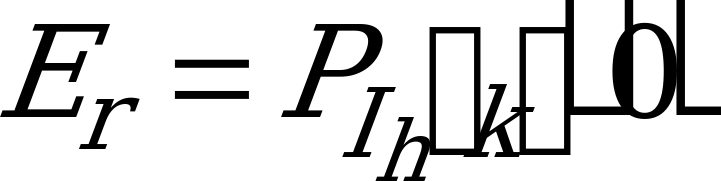(23) STEP 10 Using the AFLAT recursion outlined, the residual error due to each vector from the prequantizer at the k-th segment is evaluated, the four subsets of quantizer vectors to be searched are identified, and residual error due to each quantizer vector from the selected four subsets is computed. The index of, the quantizer vector which minimized Er over all the quantizer vectors in the four subsets, is encoded with Qk bits. STEP 11 If k < 3 then the initial conditions for doing the recursion at segment k+1 need to be computed. Set j, the lattice stage index, equal to:EMBED Equation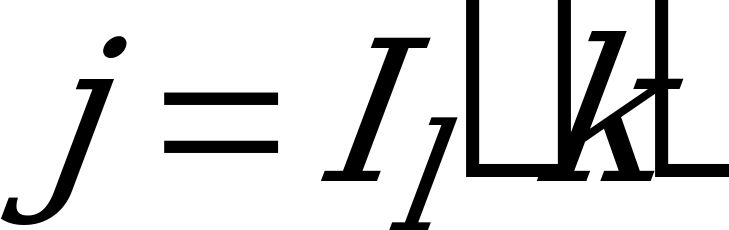(24) STEP 12 Compute: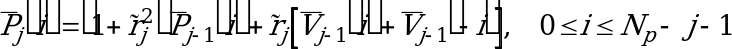(25)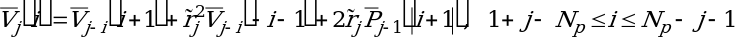(26) STEP 13 Increment j,j = j+1 STEP 14 If j £ Ih(k) go to STEP 12 STEP 15 Increment k, the vector quantizer segment index:k=k+1 STEP 16 If k £ 3 go to STEP 4.Otherwise, the indices of the reflection coefficient vectors for the three segments have been chosen, and the search of the reflection coefficient vector quantizer is terminated.

To minimize the storage requirements for the reflection coefficient vector quantizer, eight bit codes for the individual reflection coefficients are stored in the vector quantizer table, instead of the actual reflection coefficient values. The codes are used to look up the values of the reflection coefficients from a scalar quantization table with 256 entries.